# R S Aggarwal Solutions for Class 11 Maths Chapter 4- Principle of Mathematical Induction

R S Aggarwal Solutions are considered to be very useful when you are preparing for the Class 11 Maths exams. Here, we bring to you the R S Aggarwal Solutions for Class 11 Maths, providing the detailed explanations to the questions present in the exercises of Chapter 4- Principle of Mathematical Induction. These answers have been devised by the subject matter experts at BYJU’S.

The complete solutions of Class 11 Maths Chapter 4 – Principle of Mathematical Induction in PDF format is provided here. You can avail these R S Aggarwal solutions online or download it for free to practice them offline as well.

### Download PDF of R S Aggarwal Solutions for Class 11 Maths Chapter 4- Principle of Mathematical Induction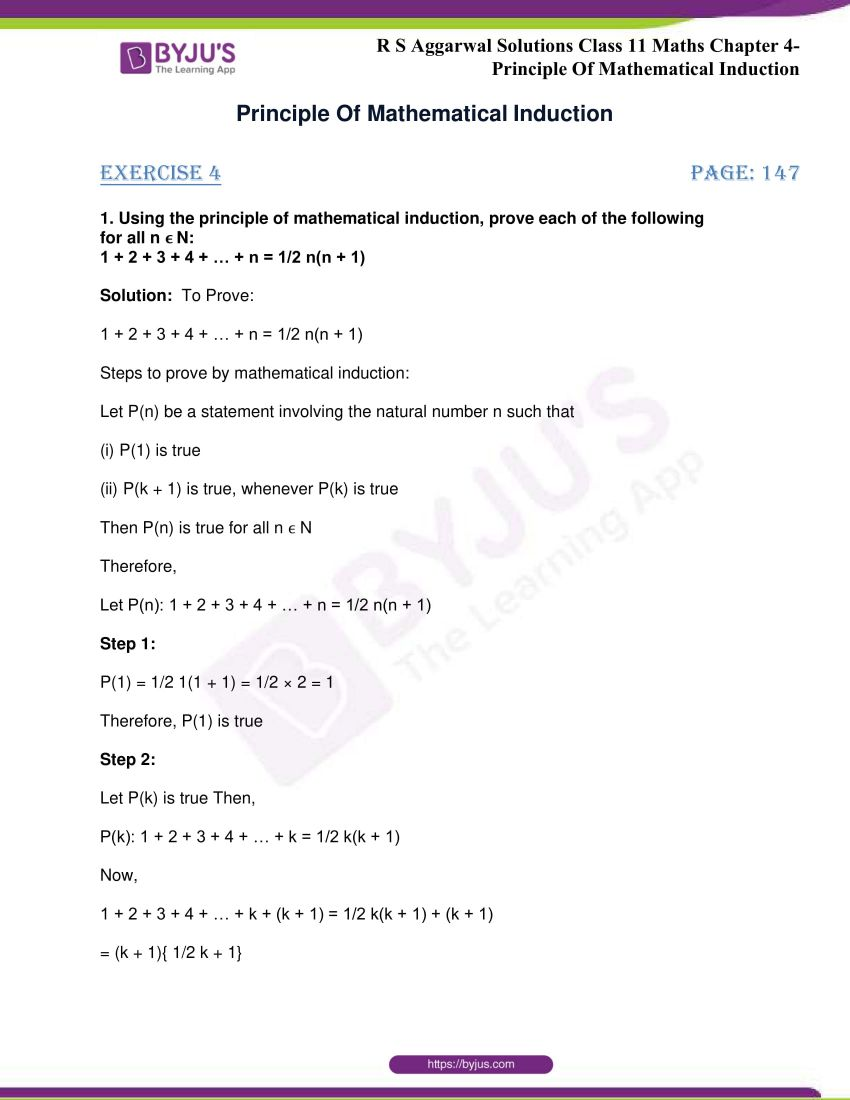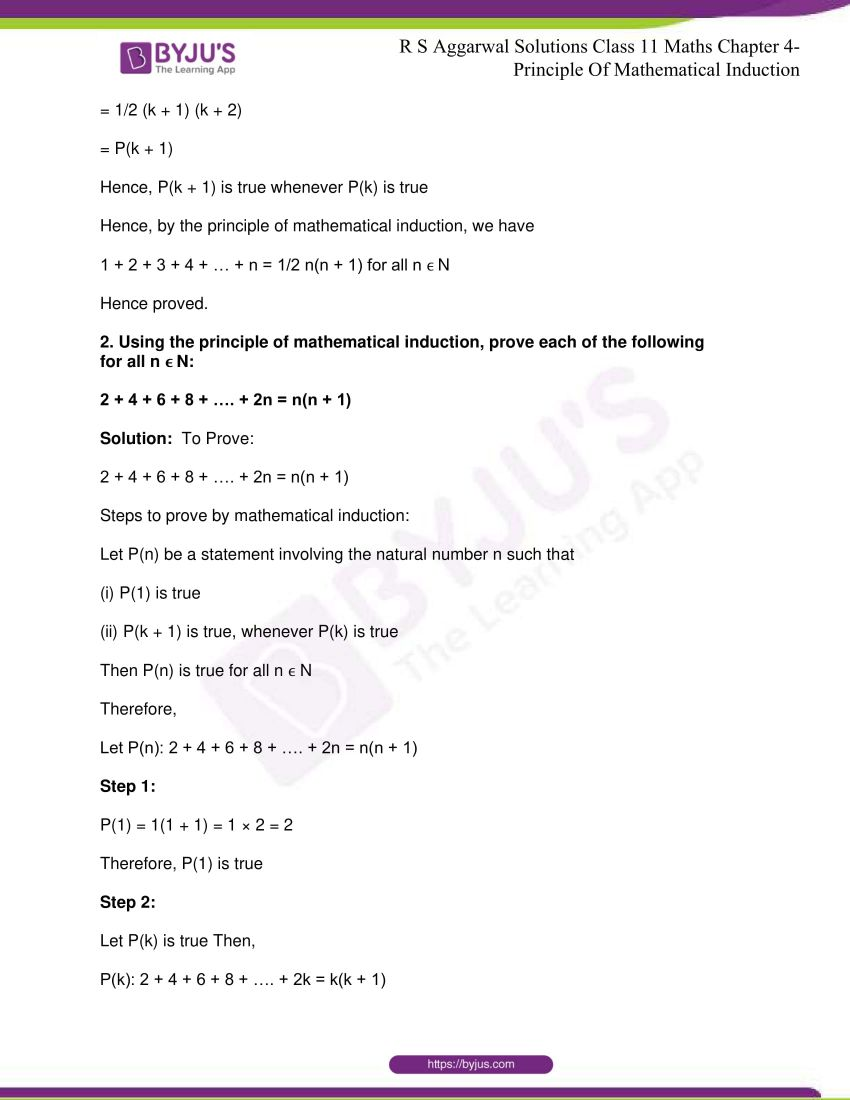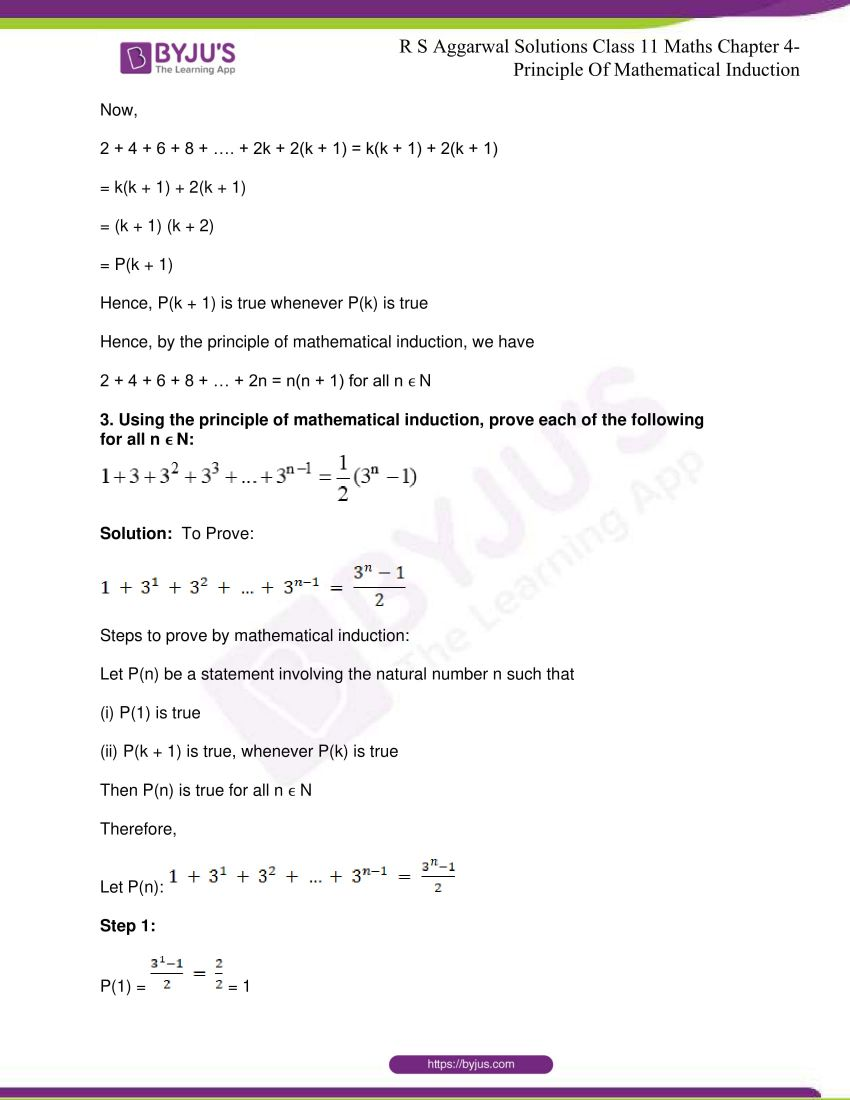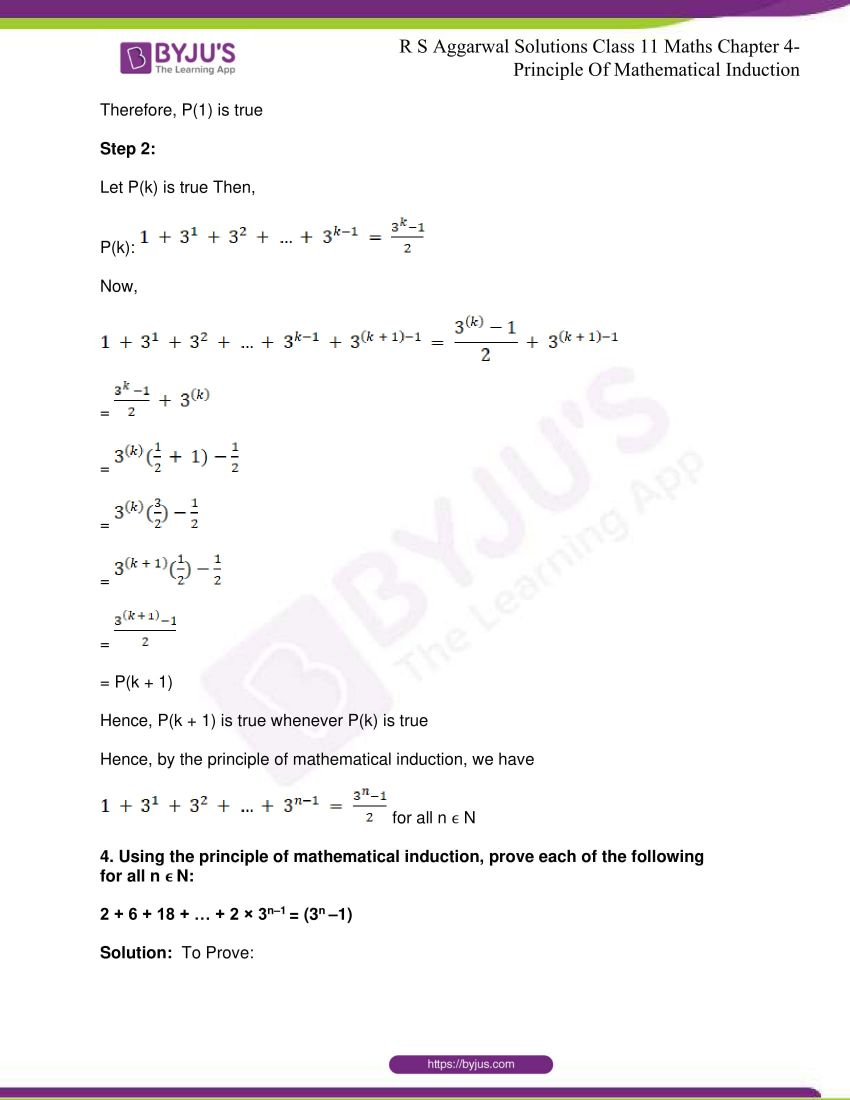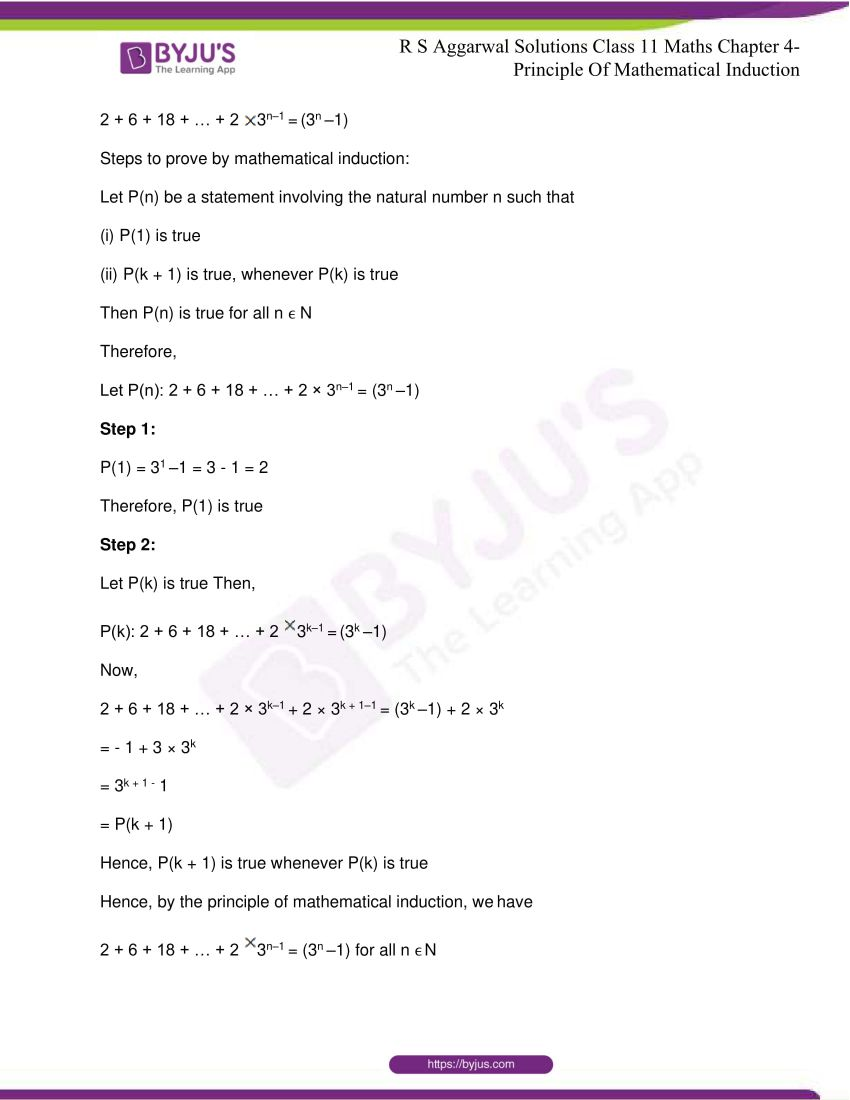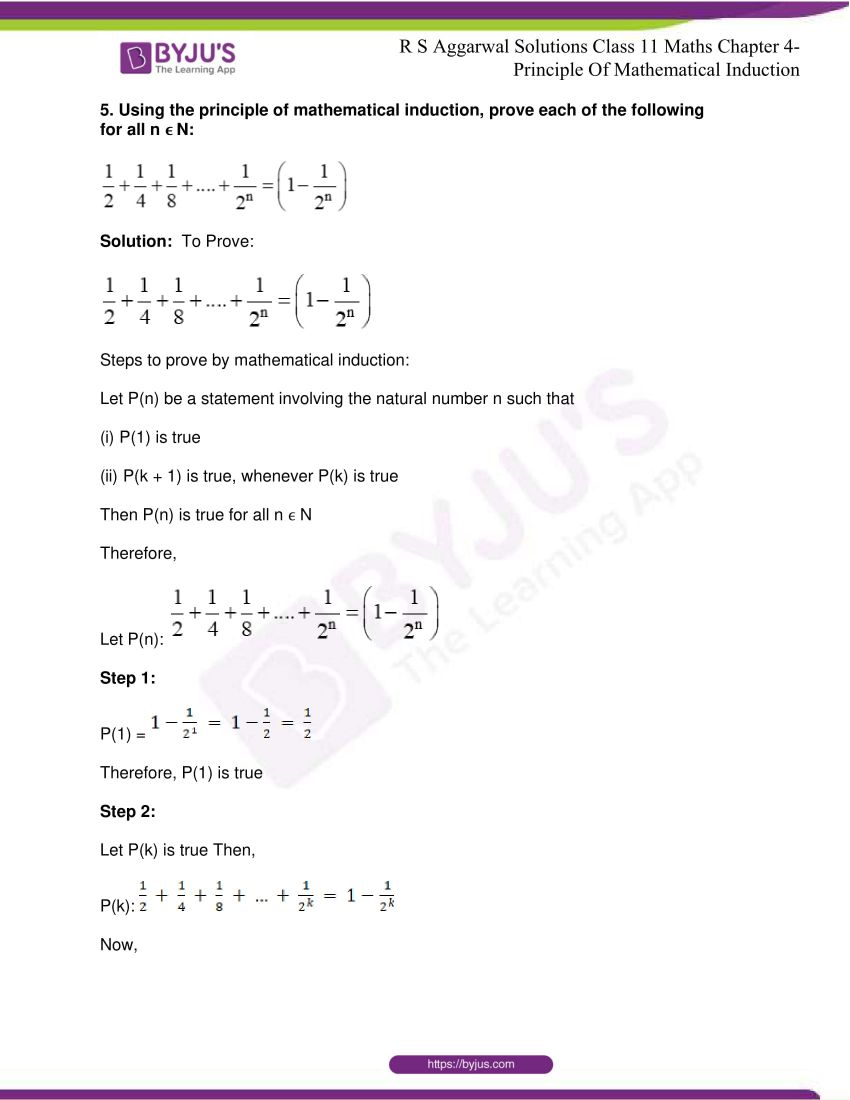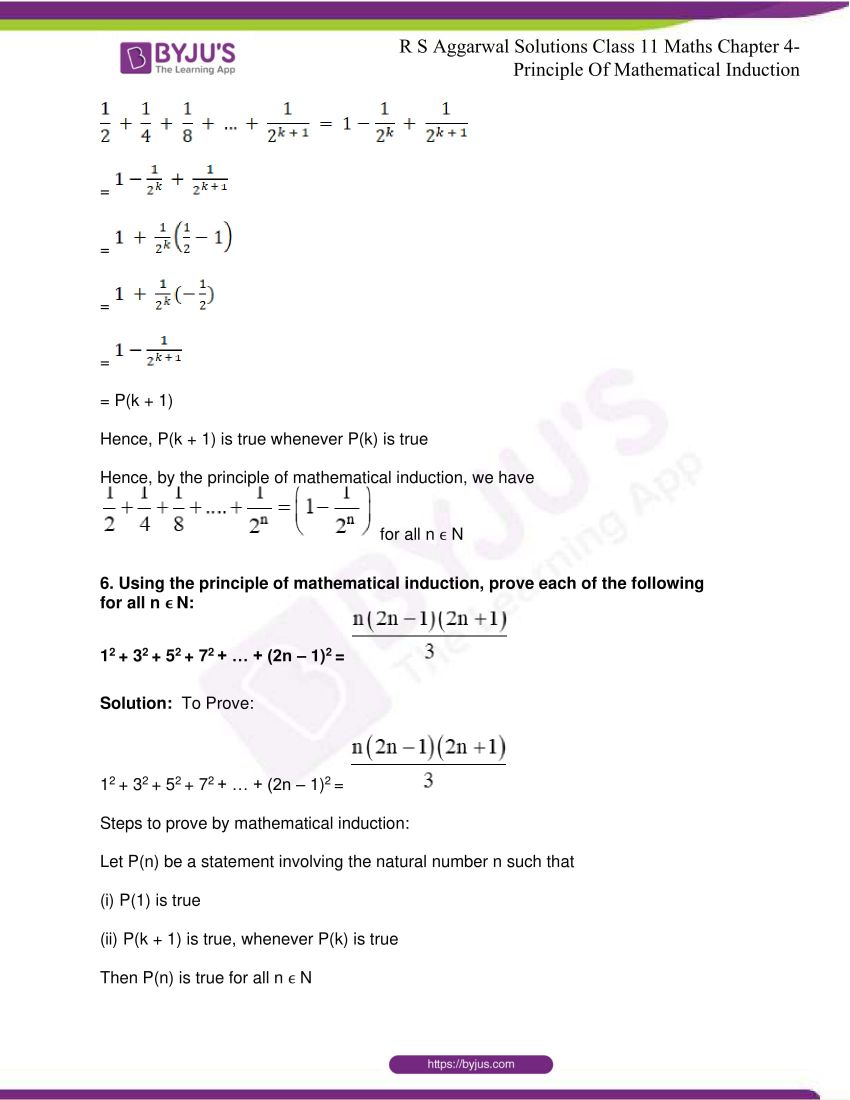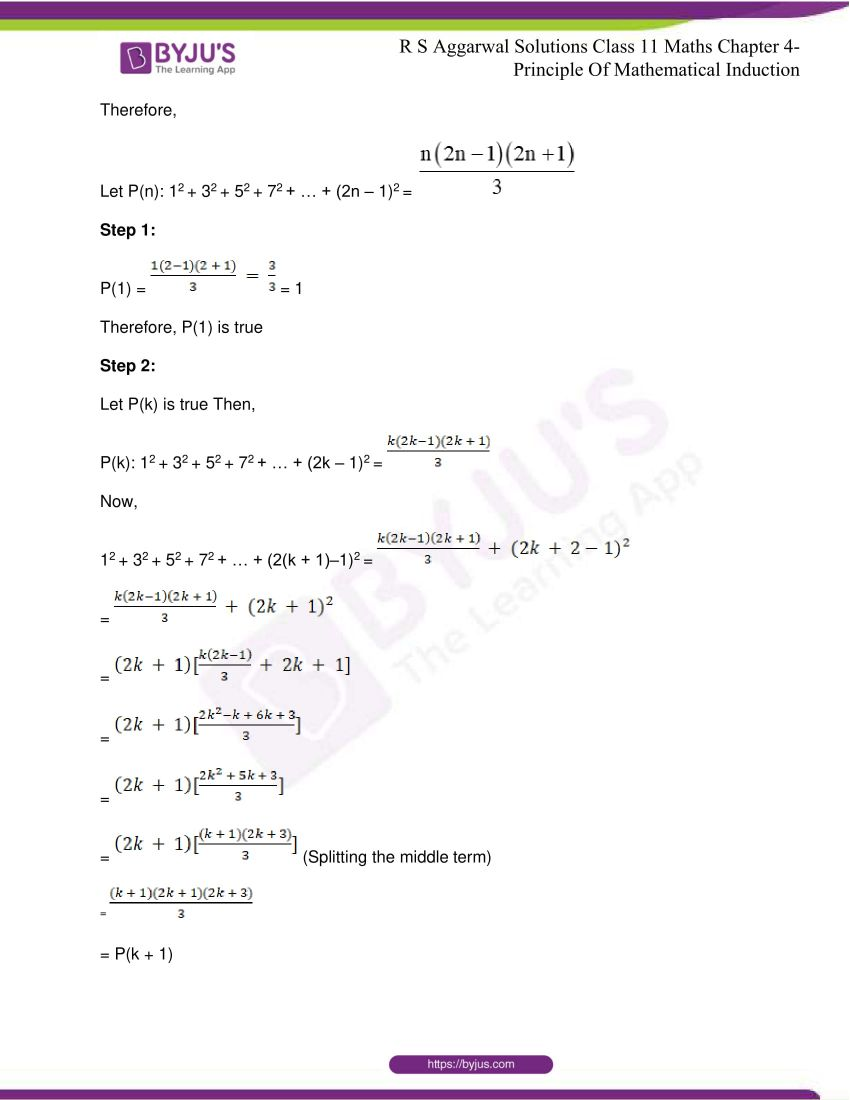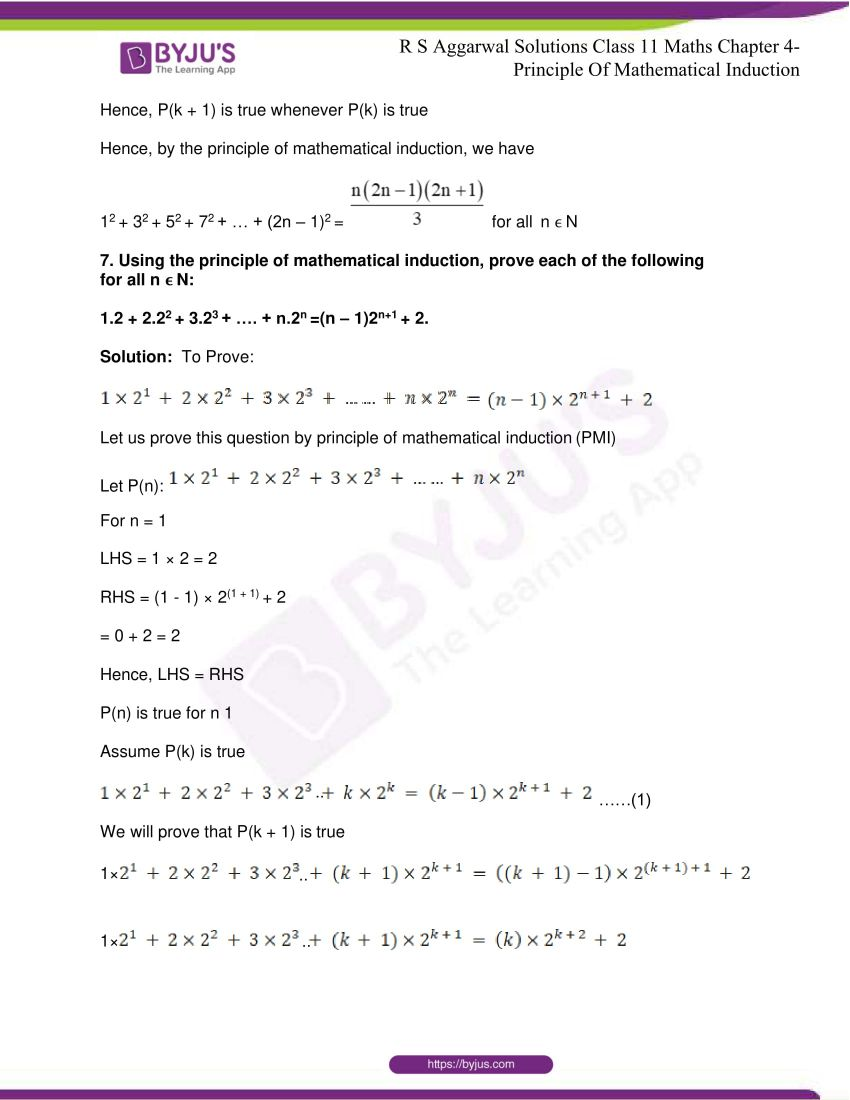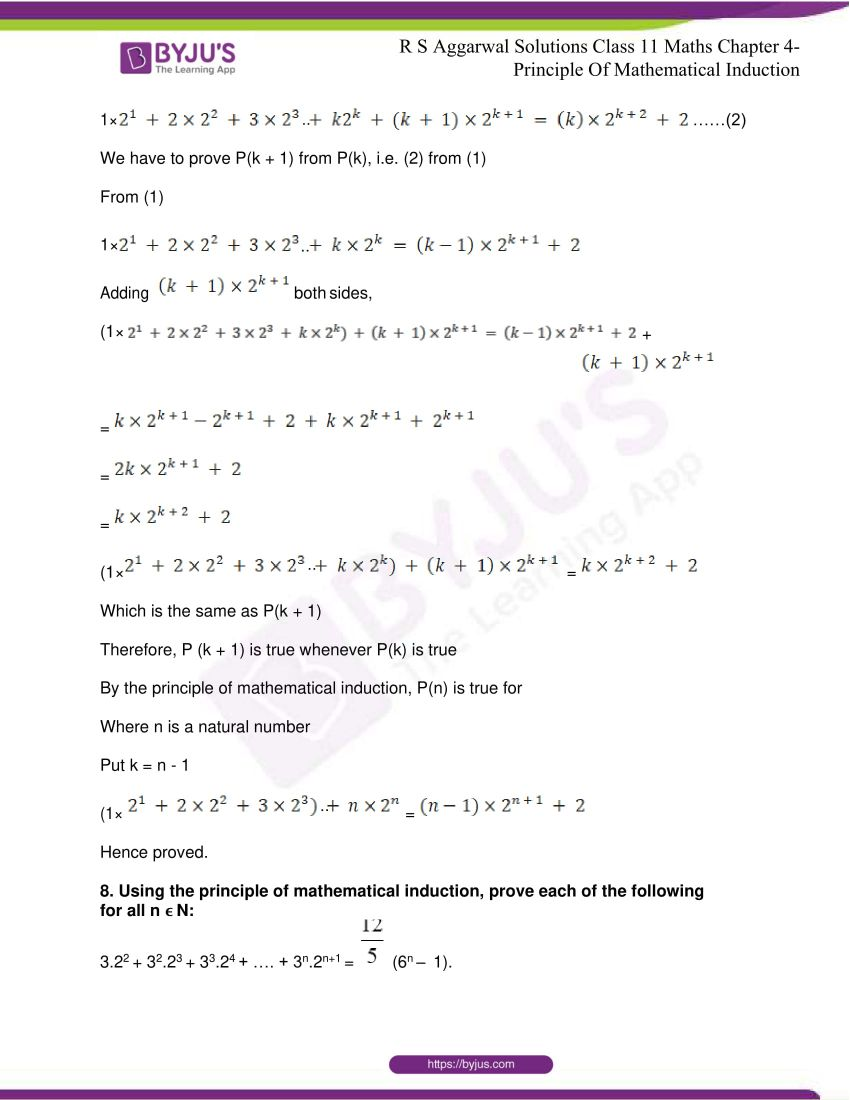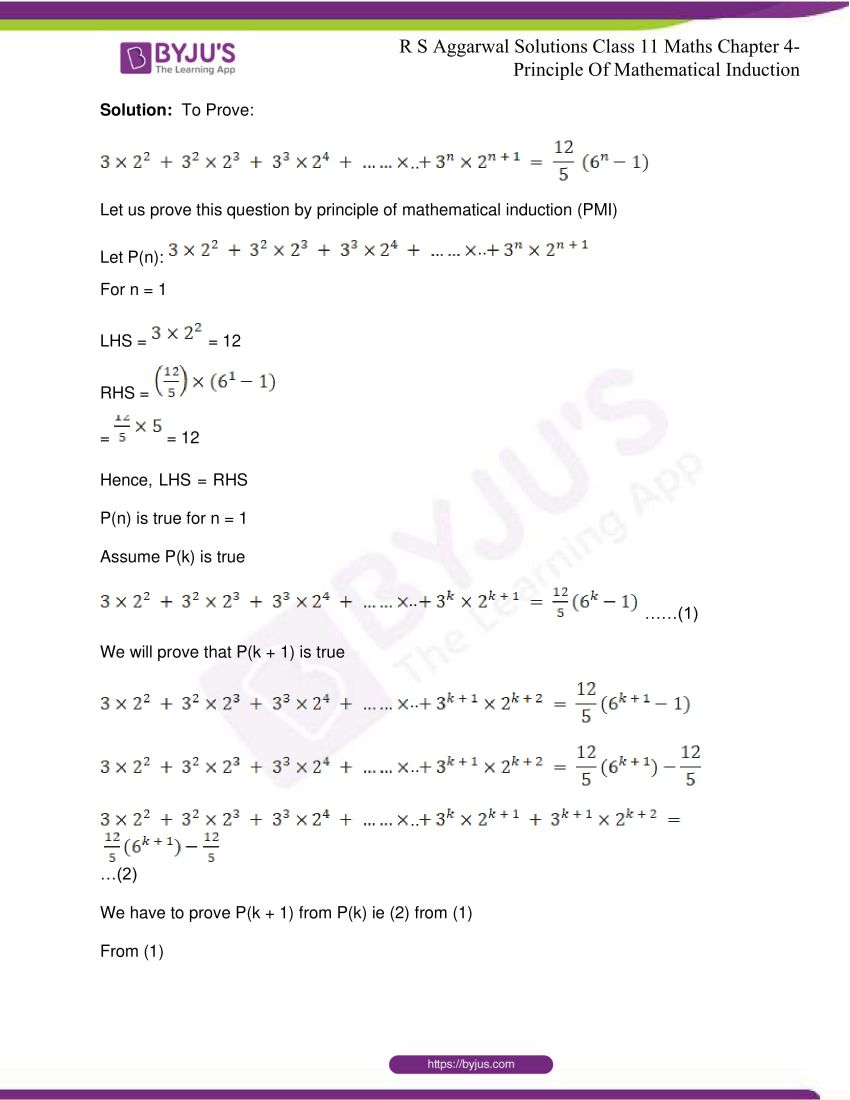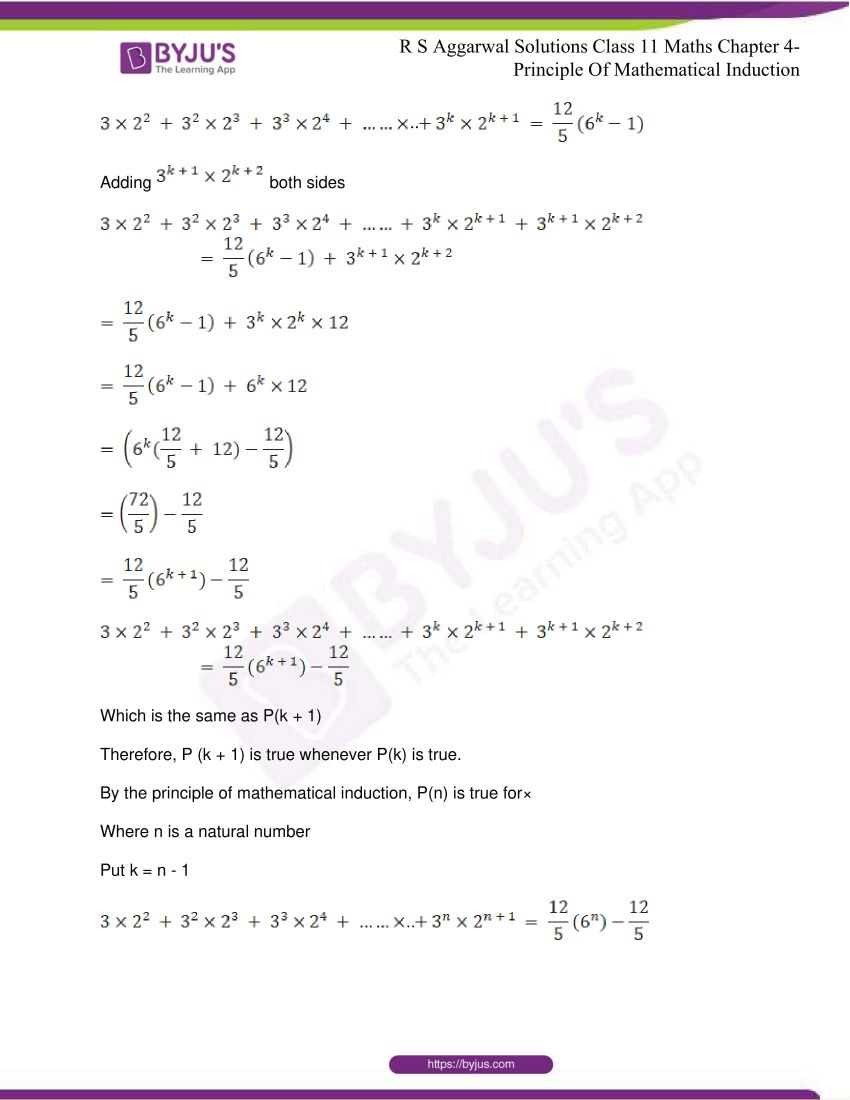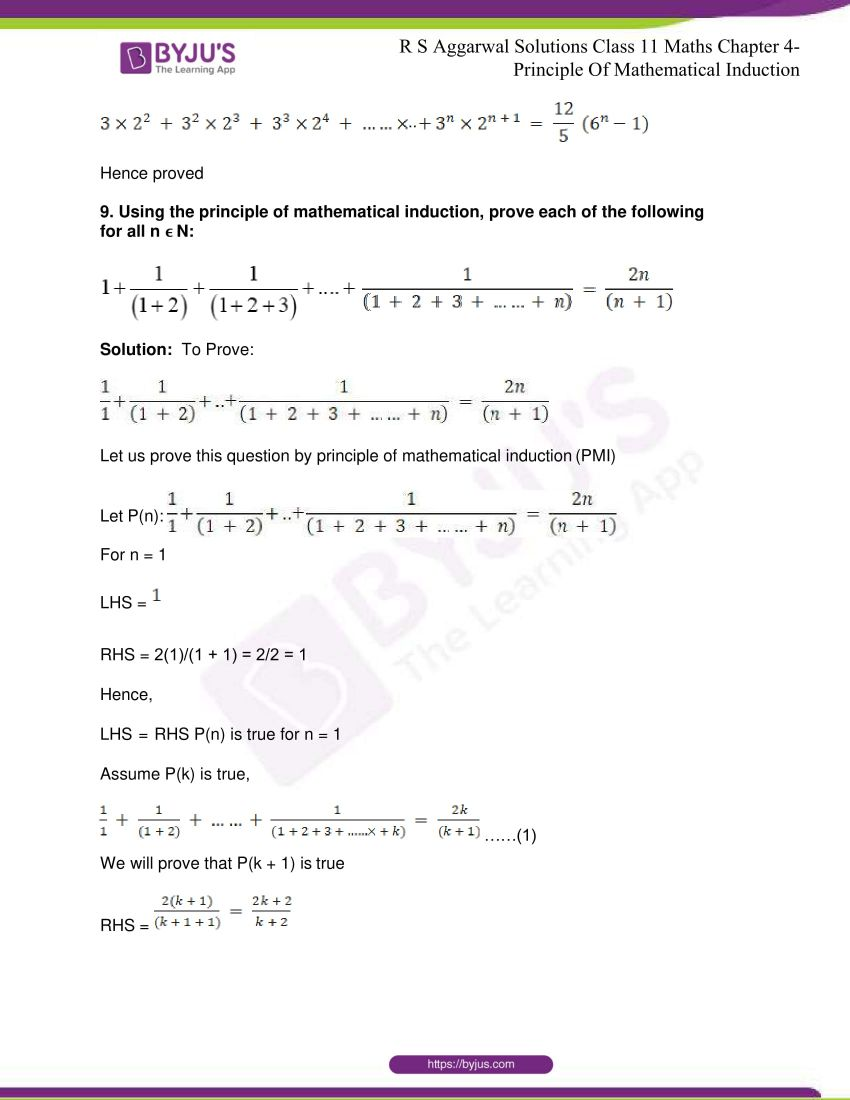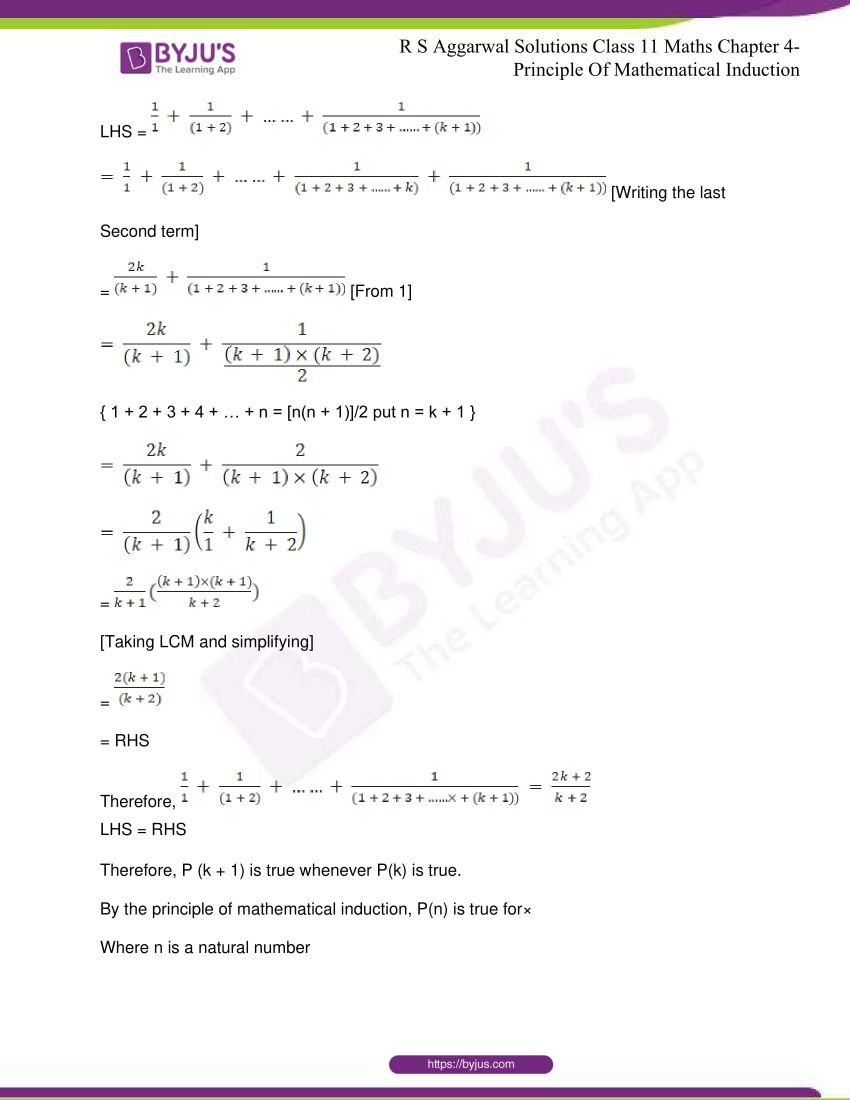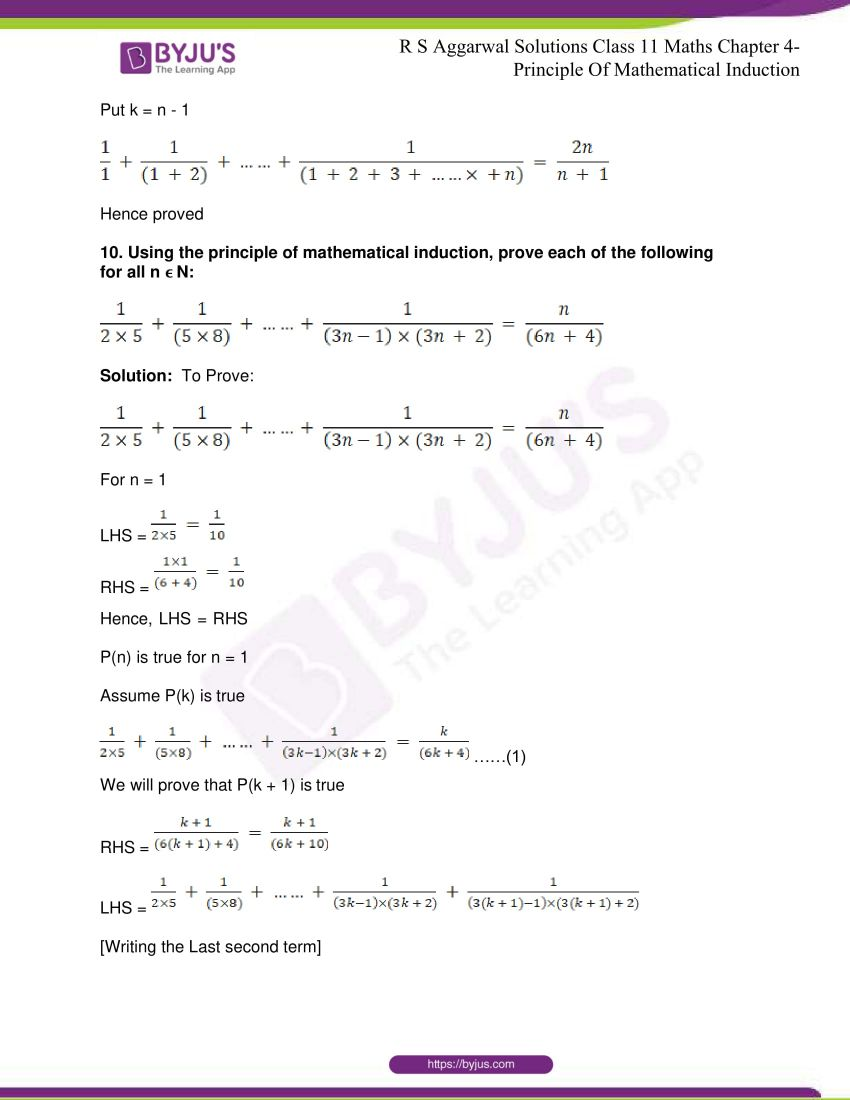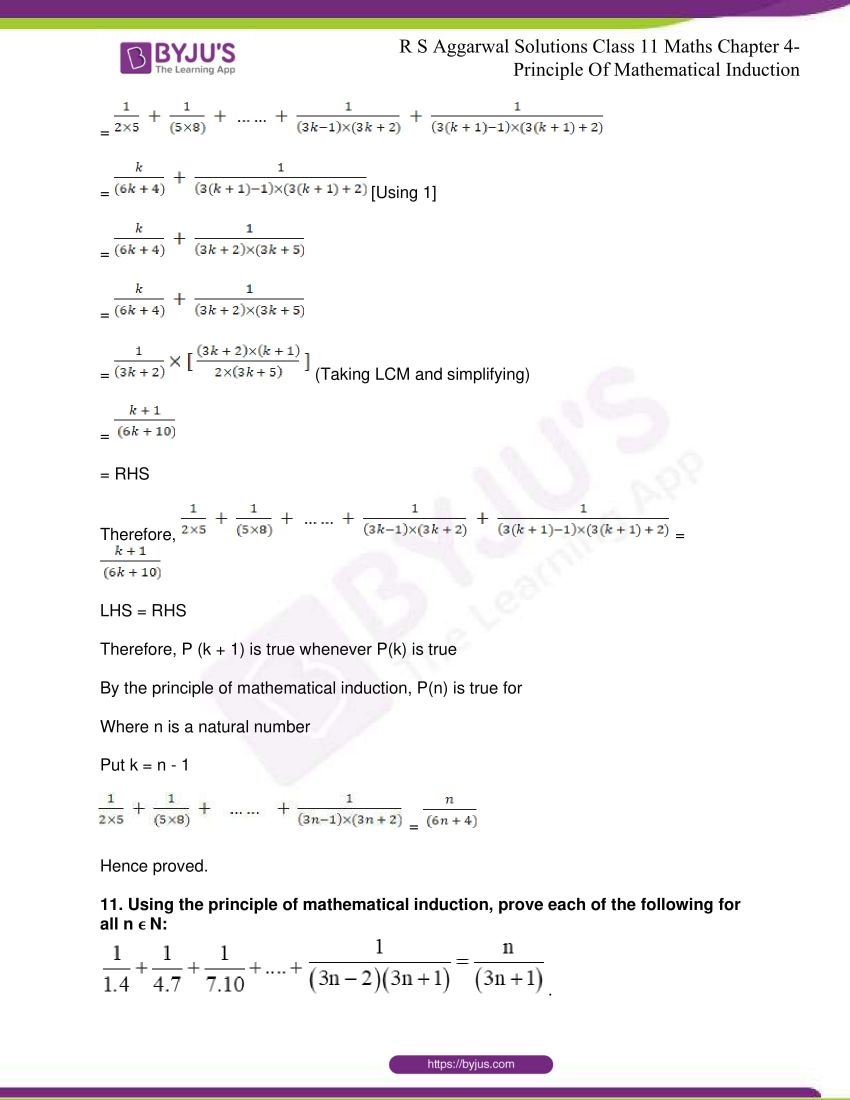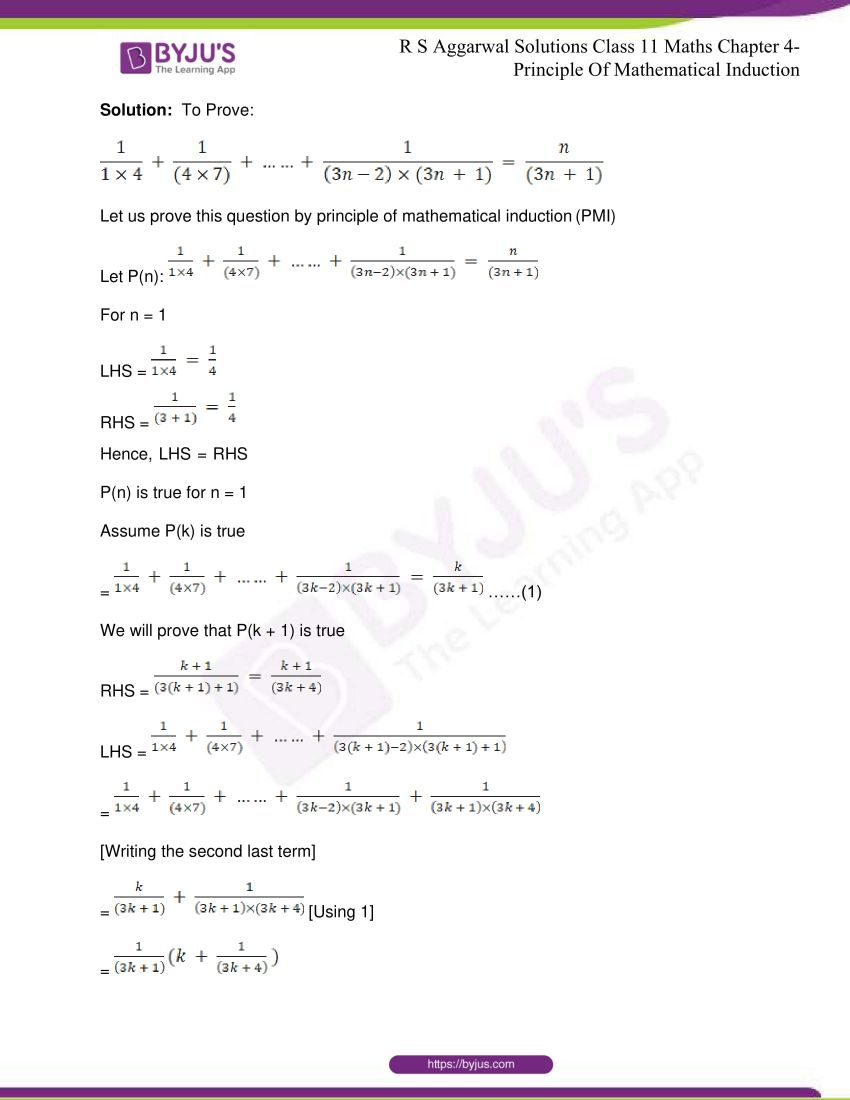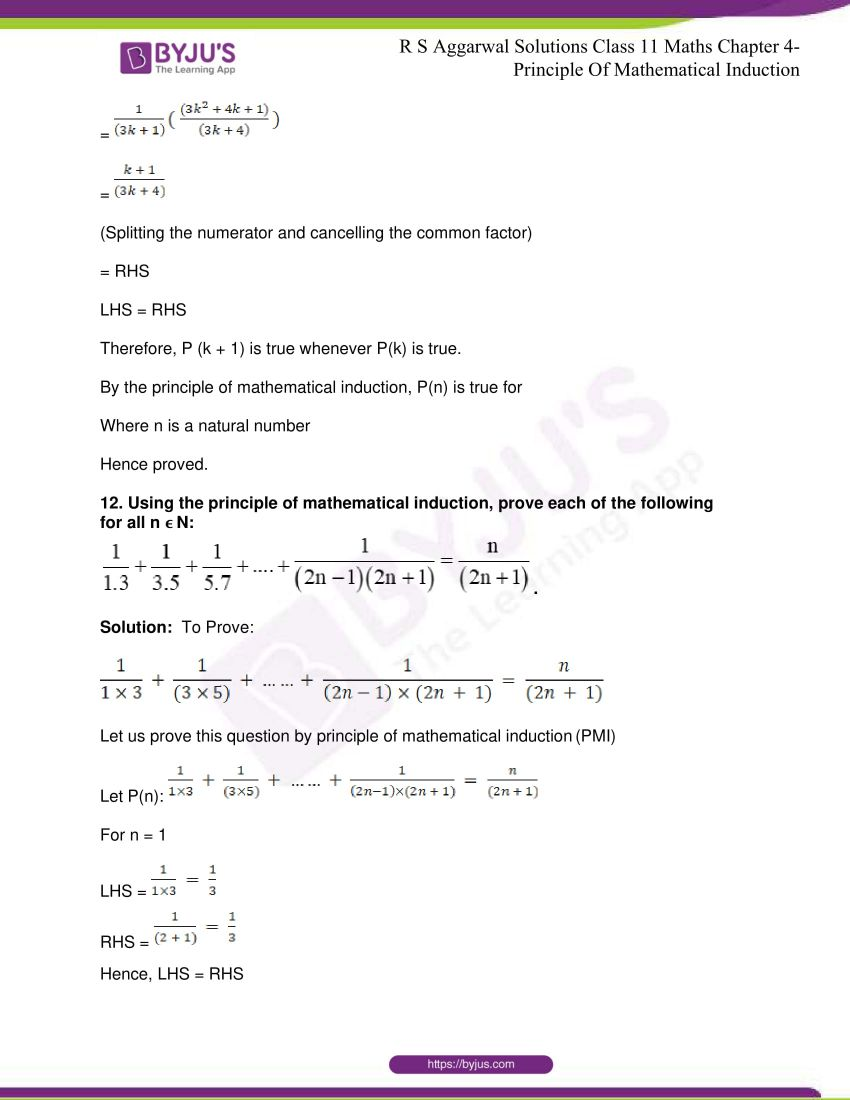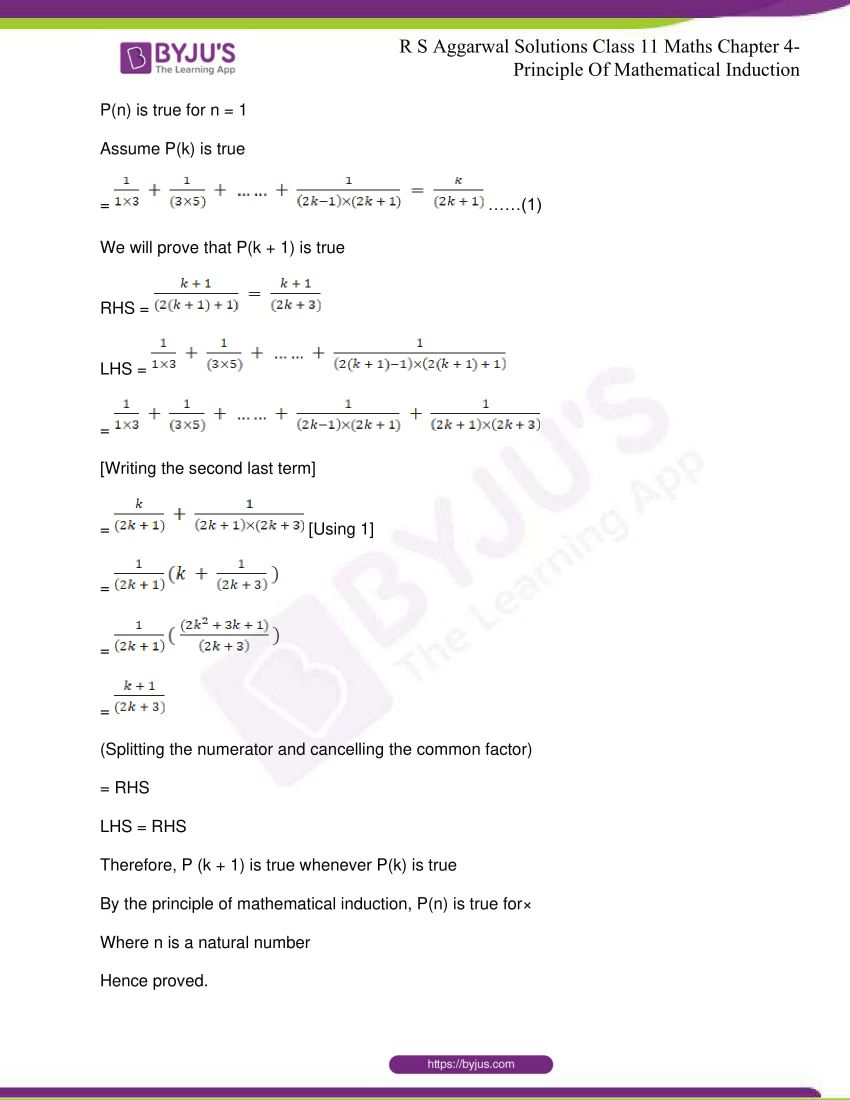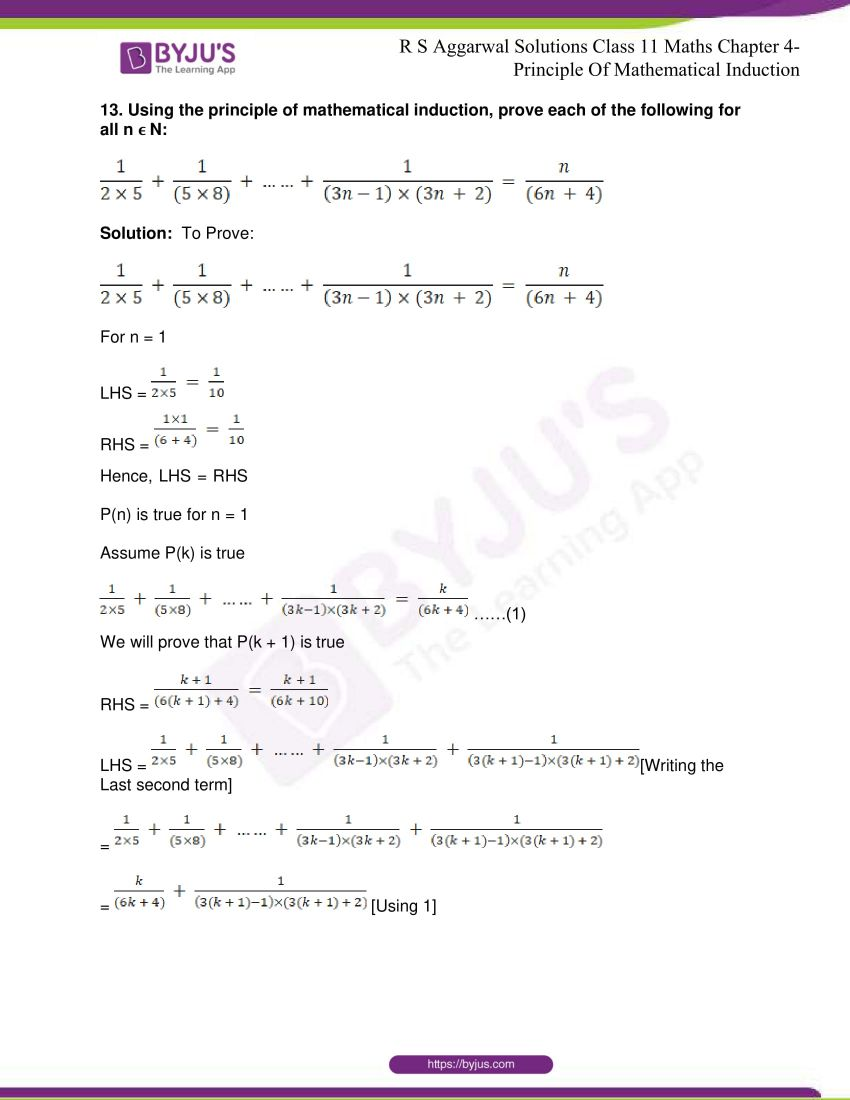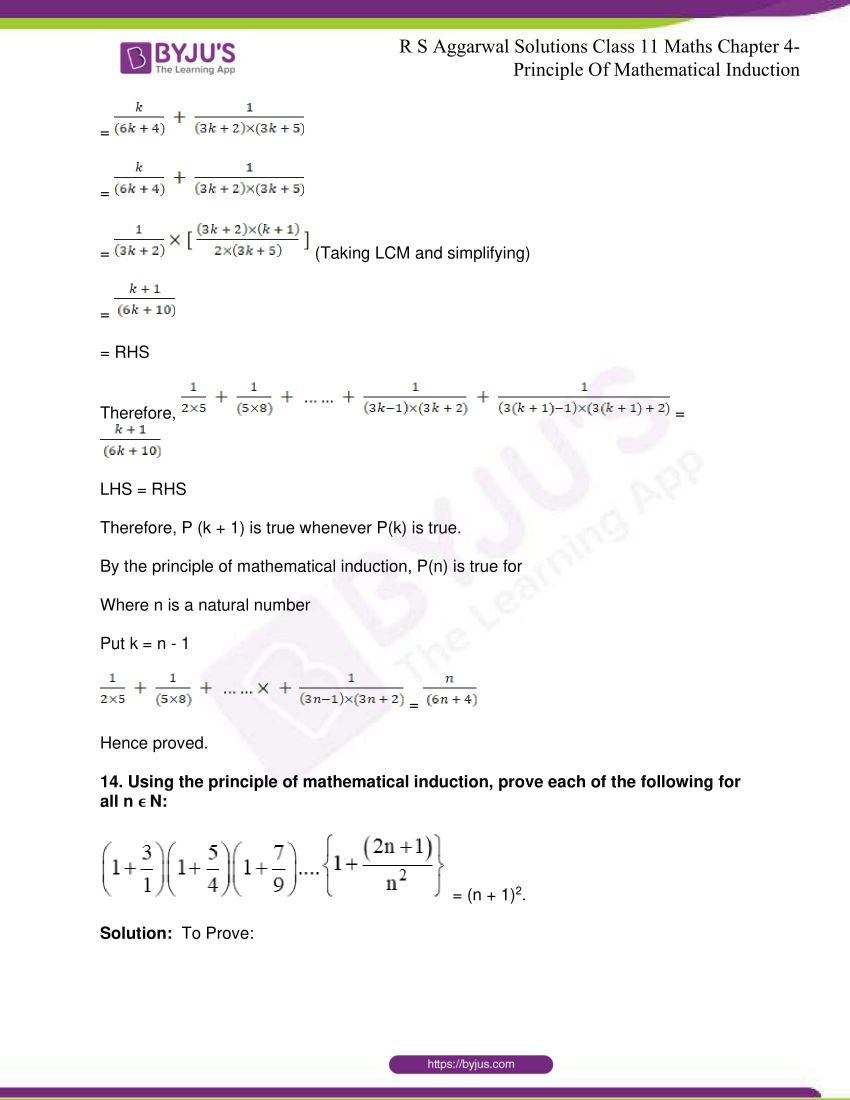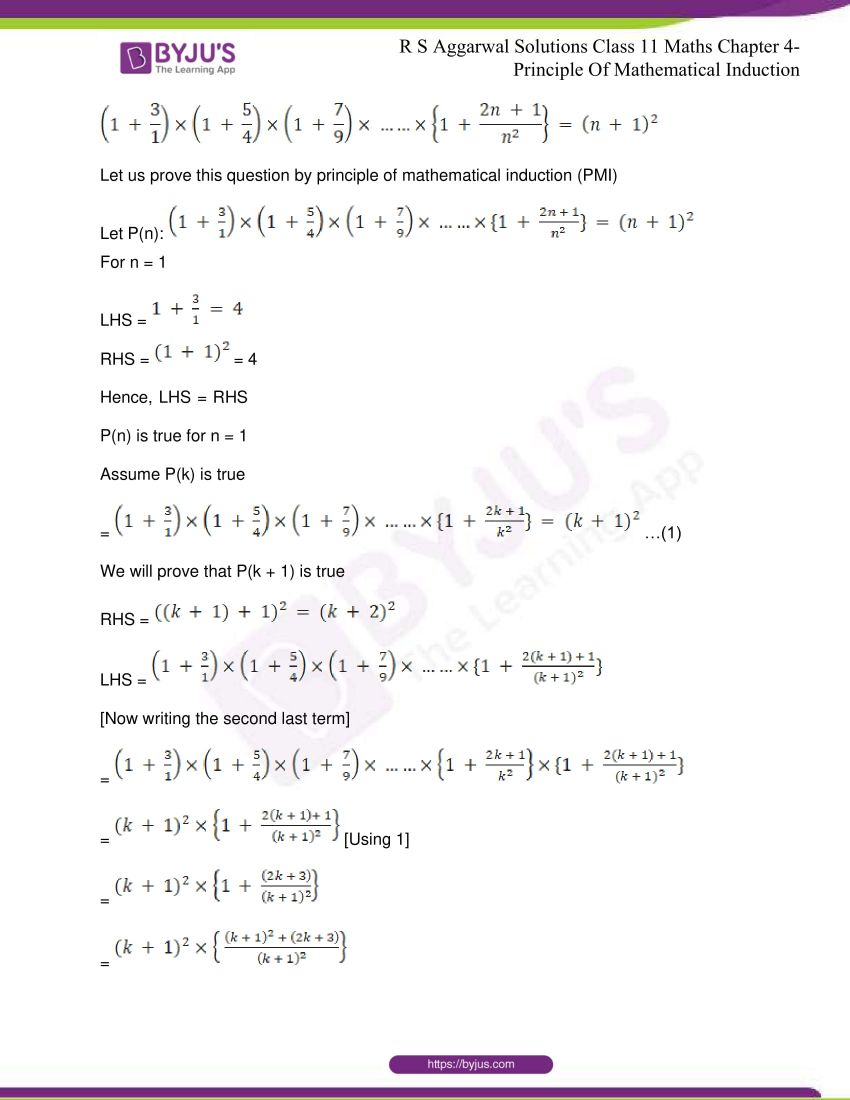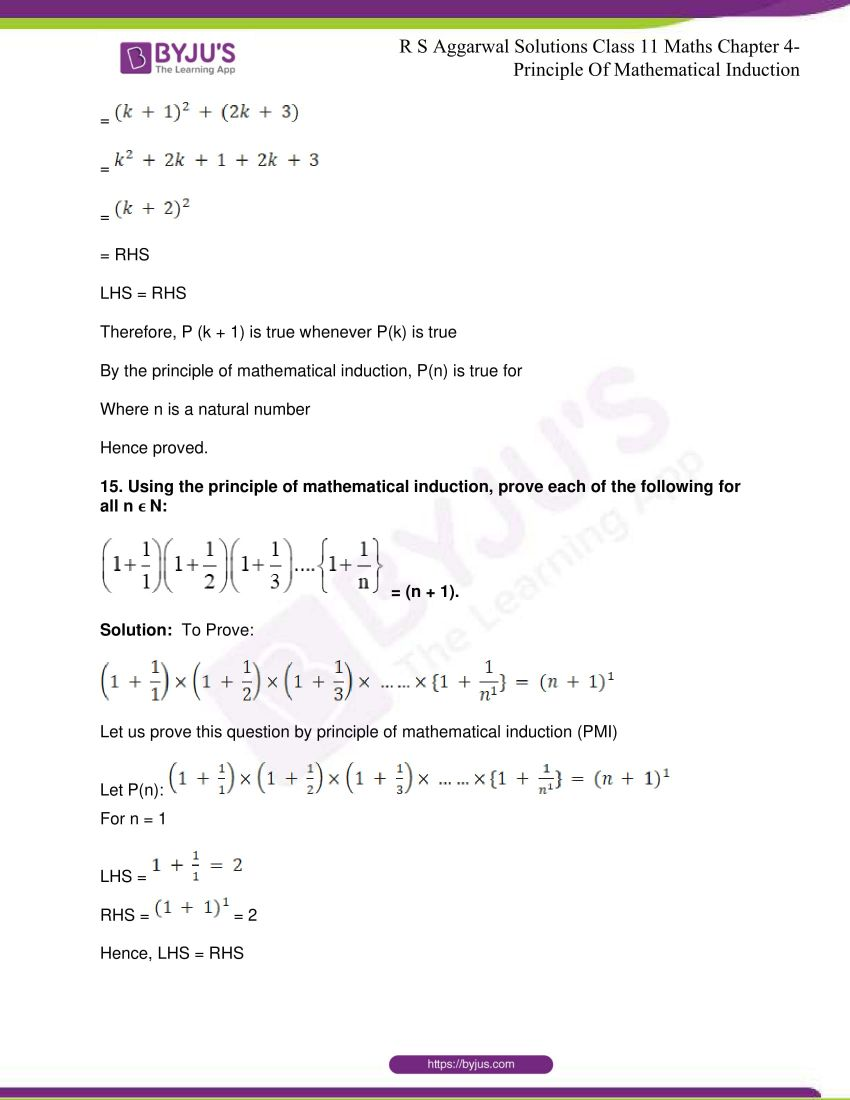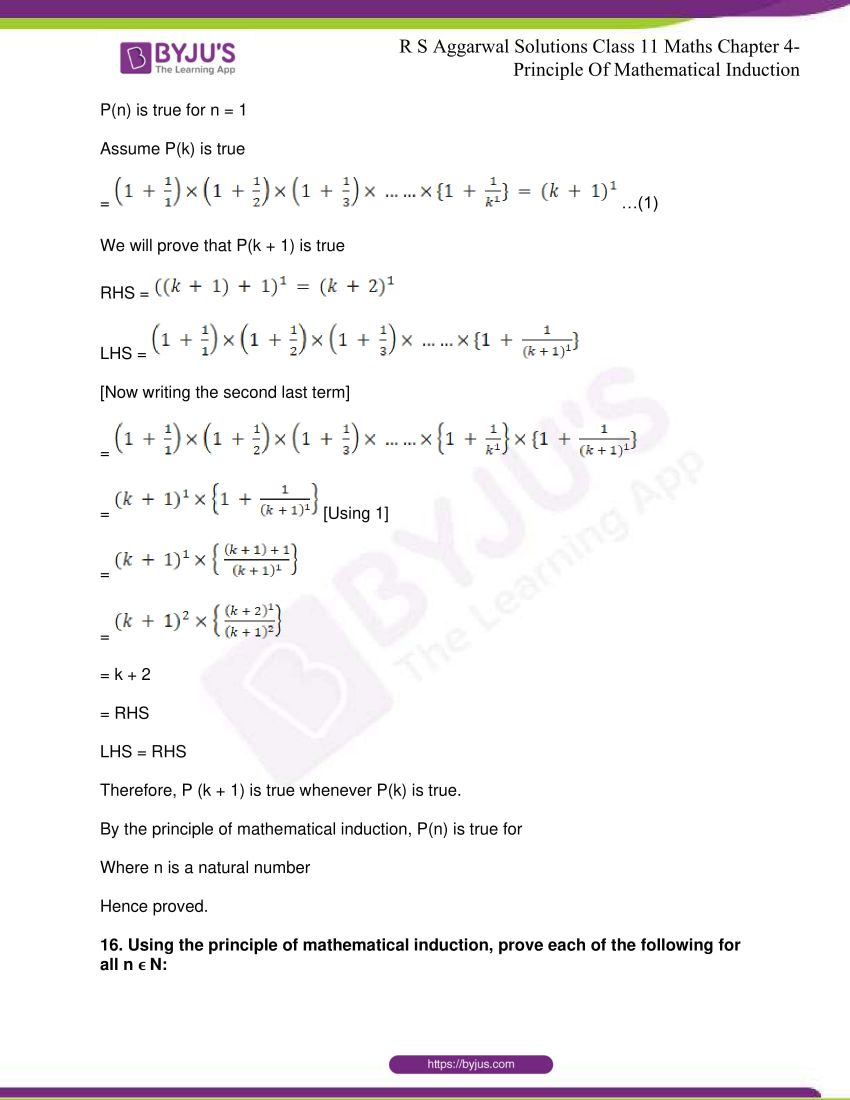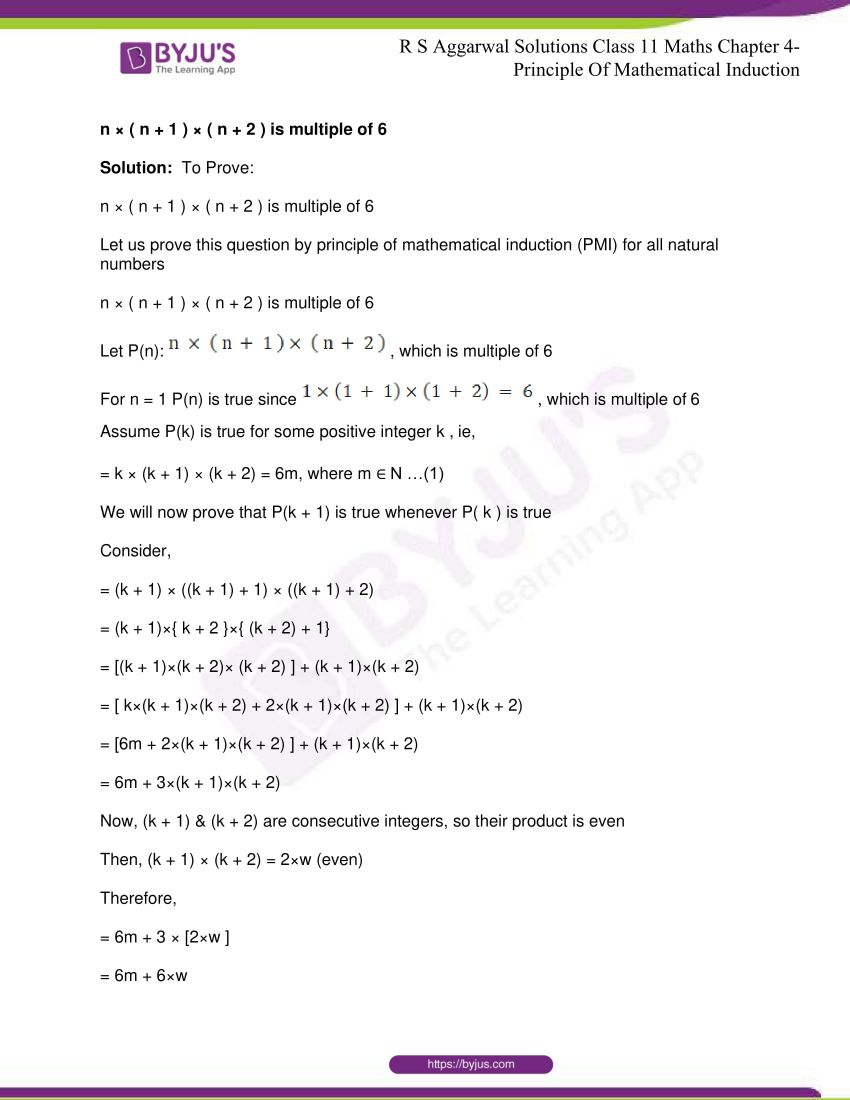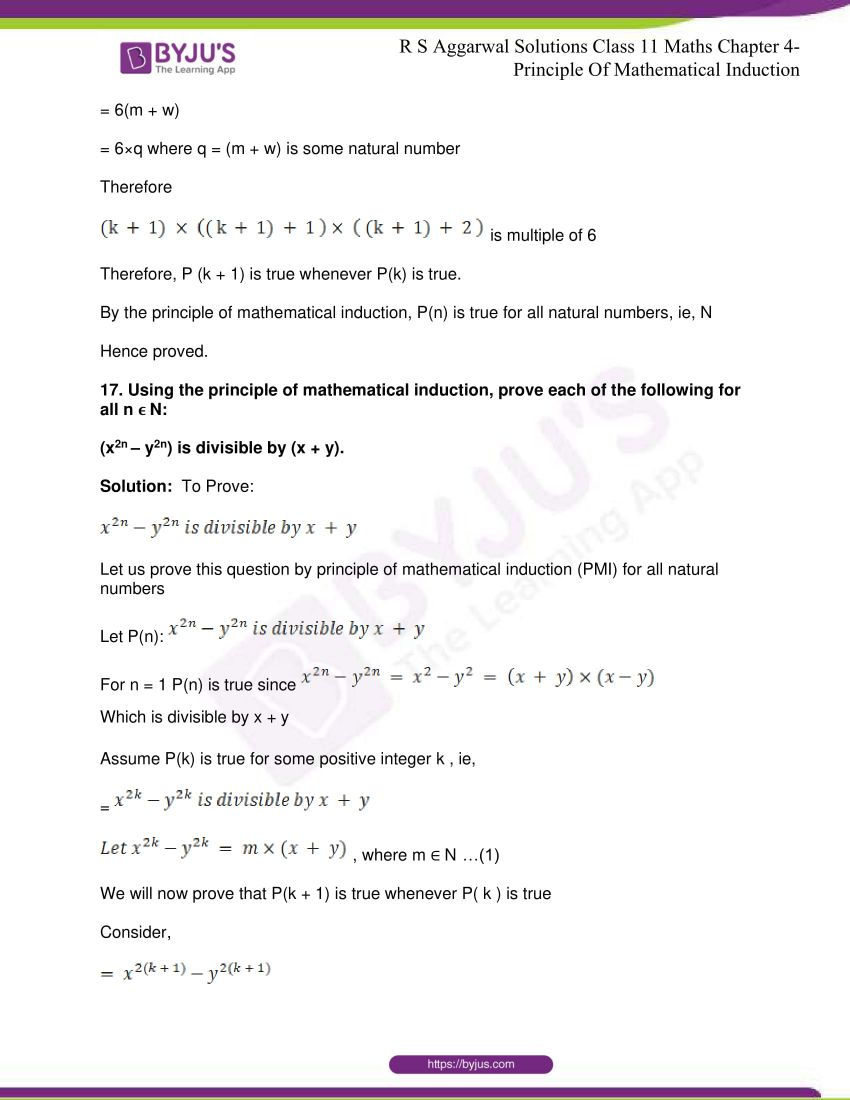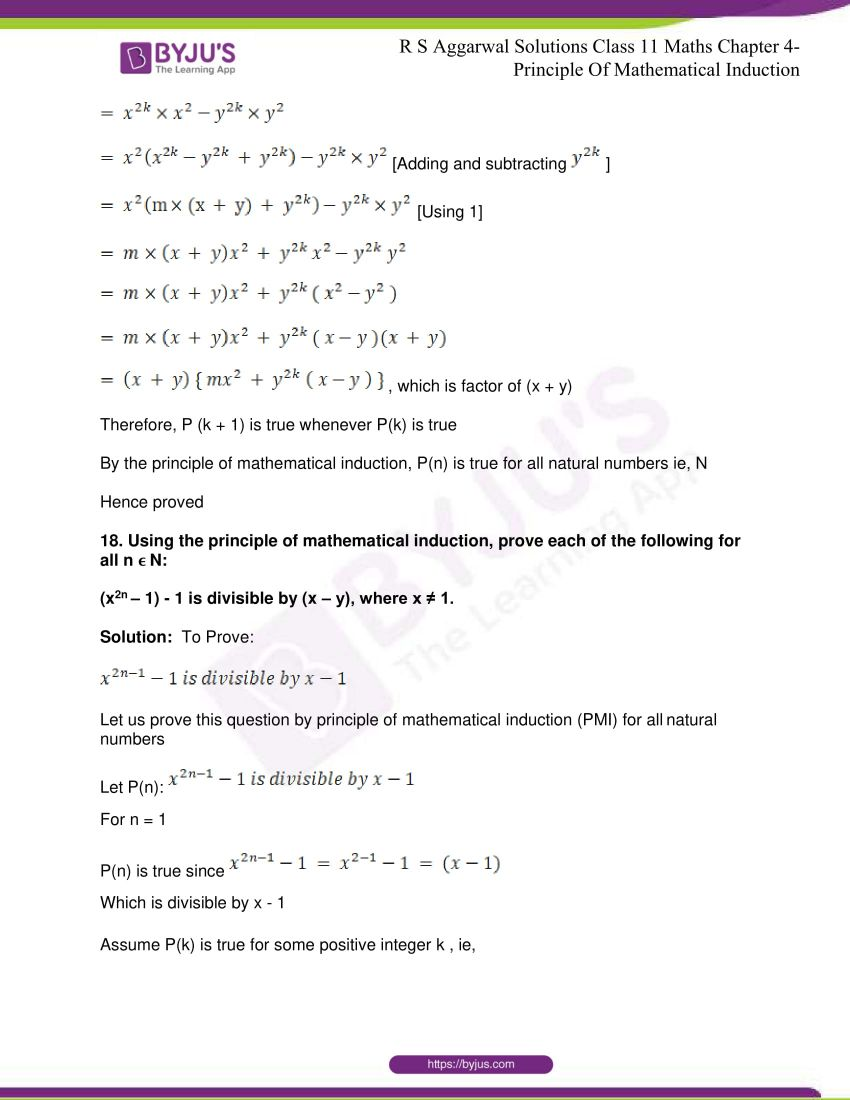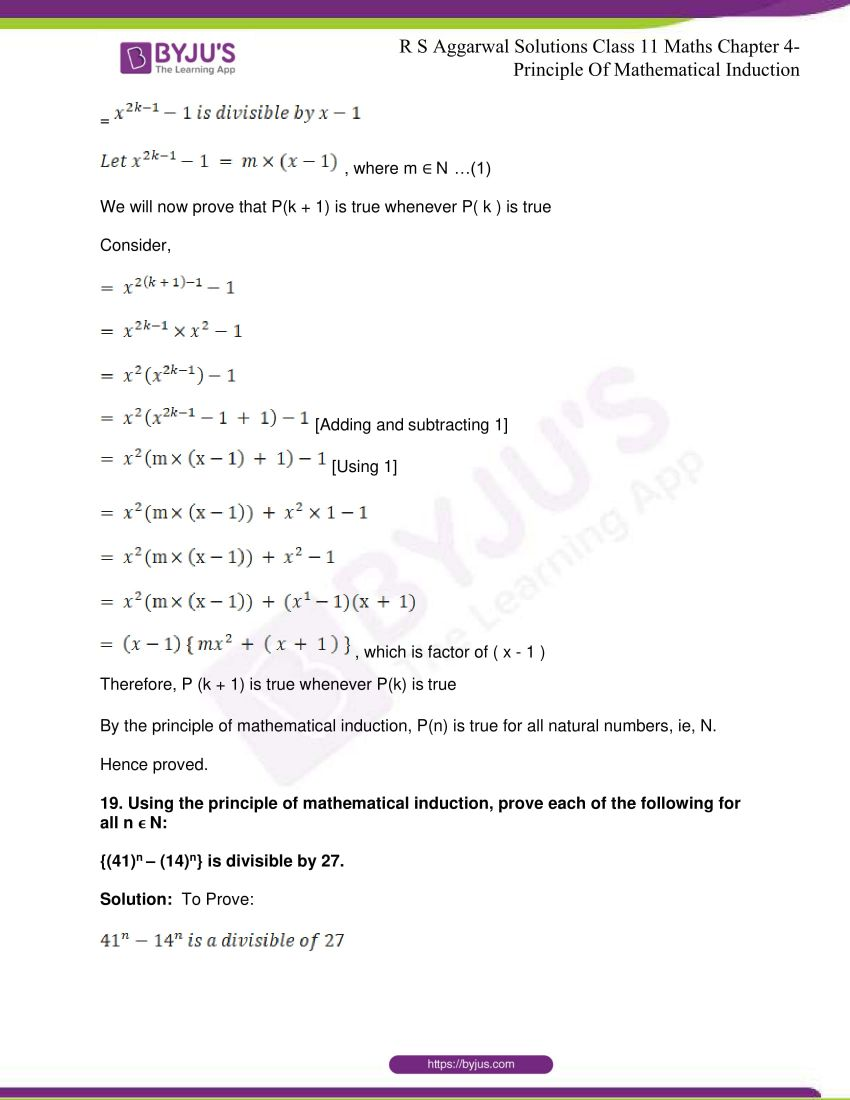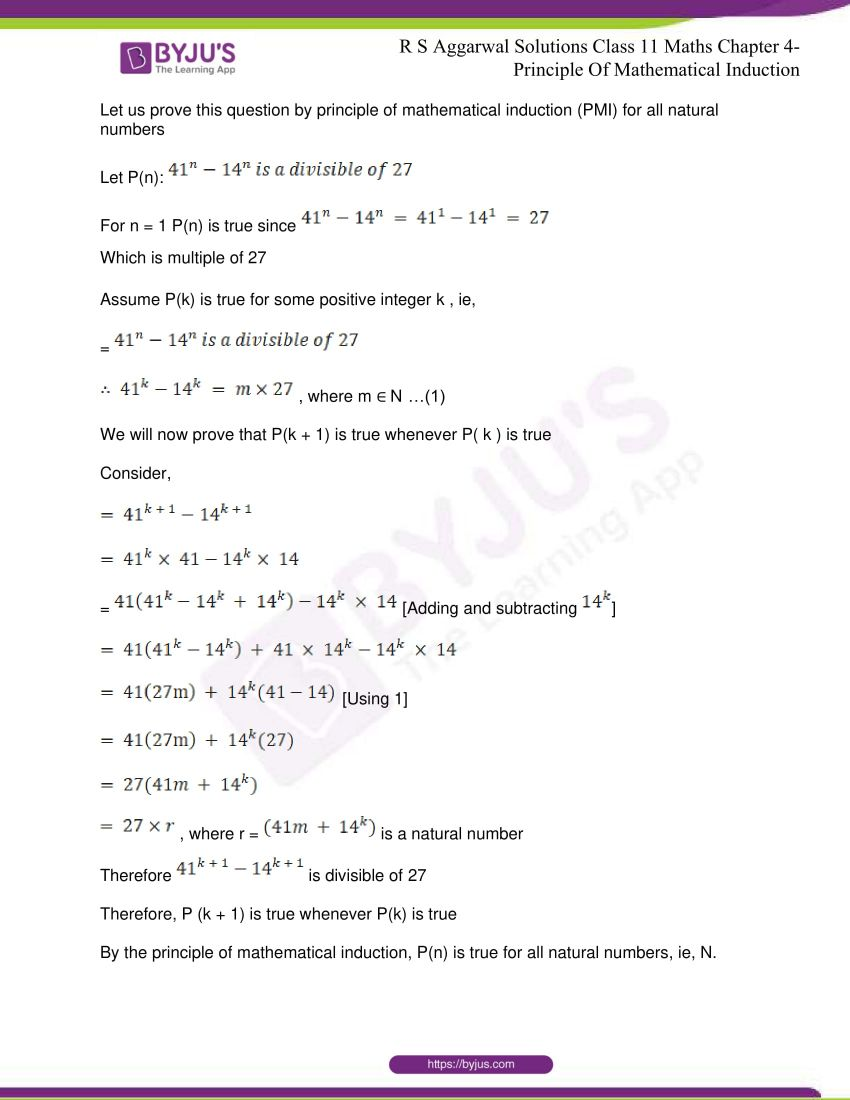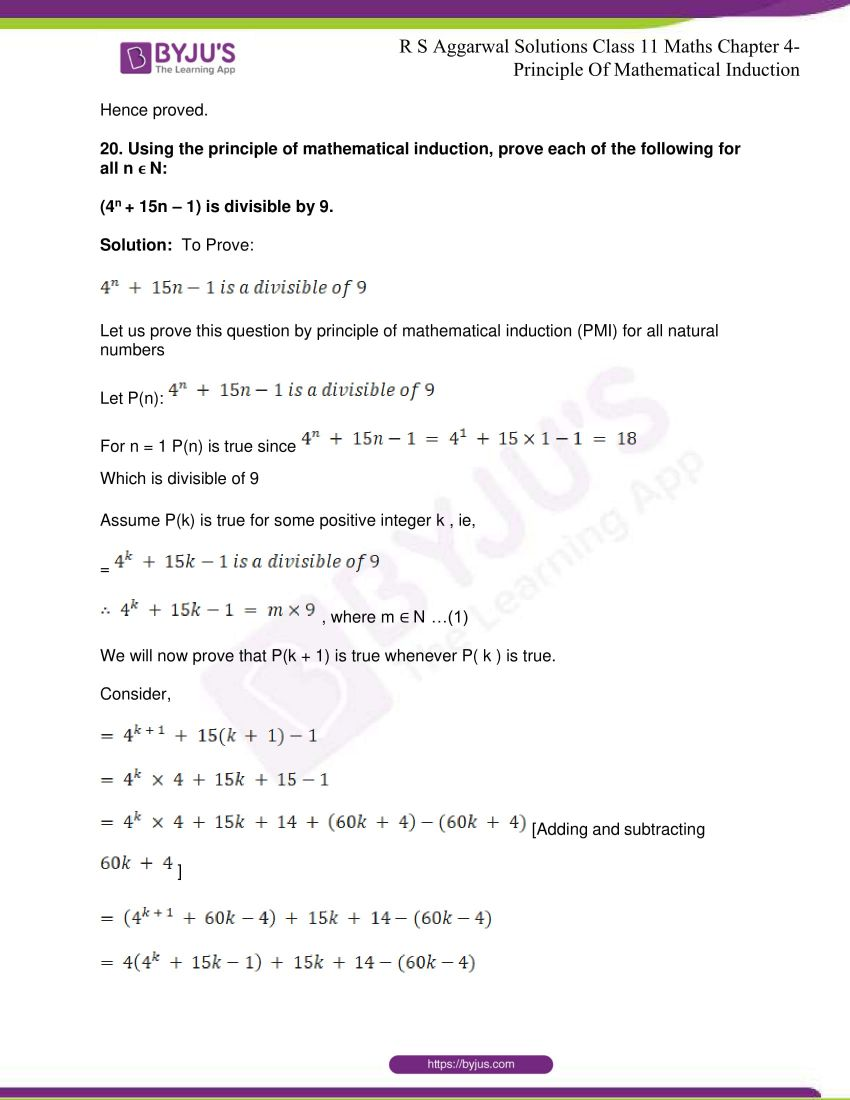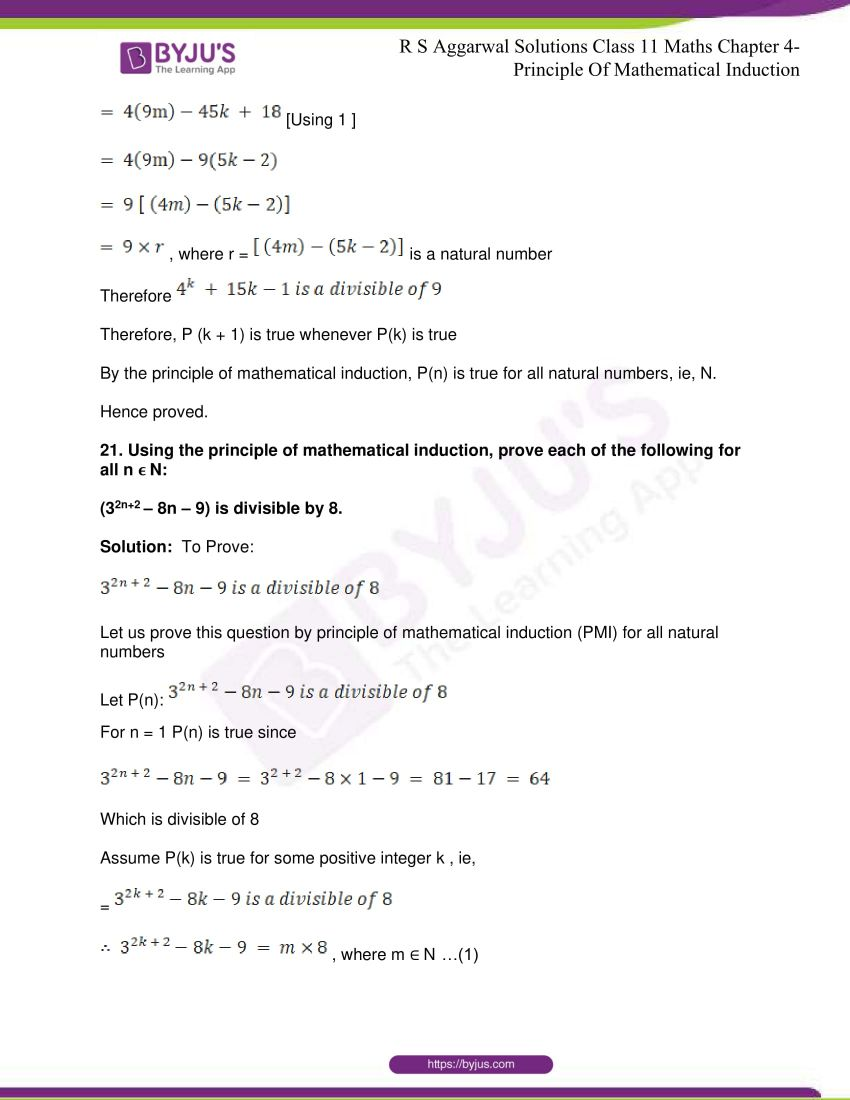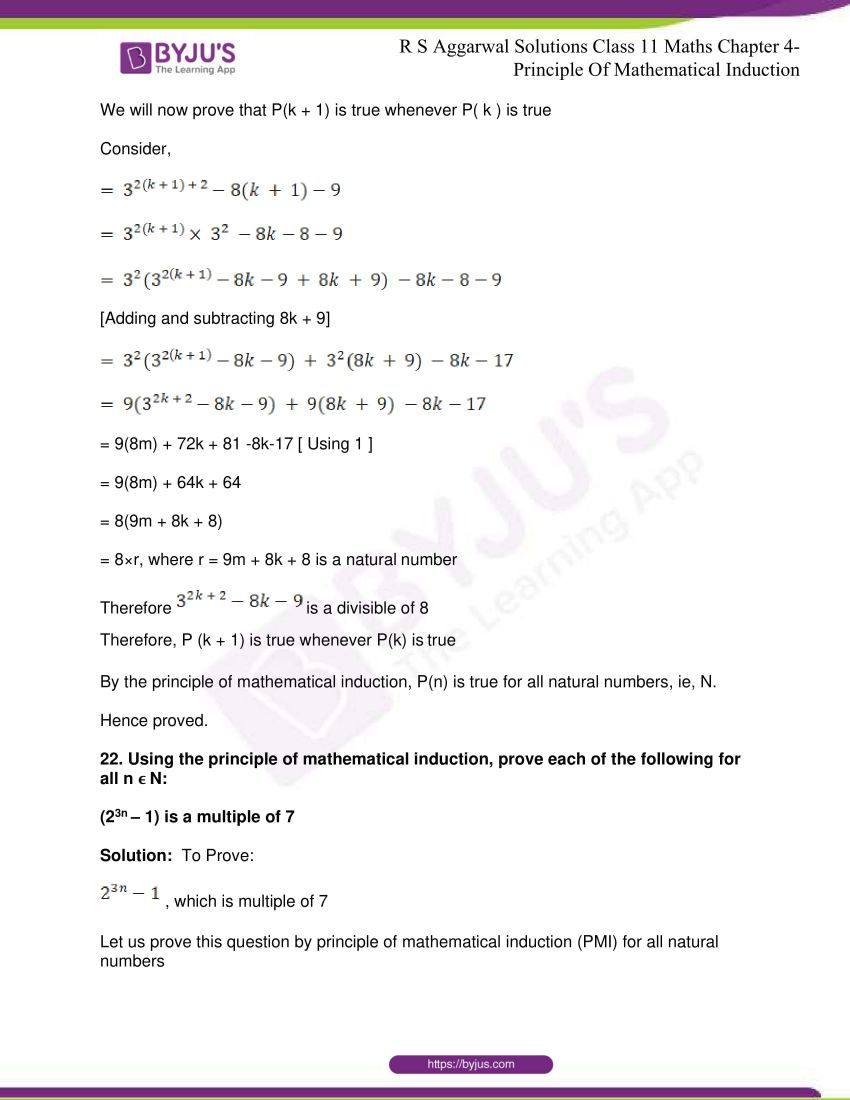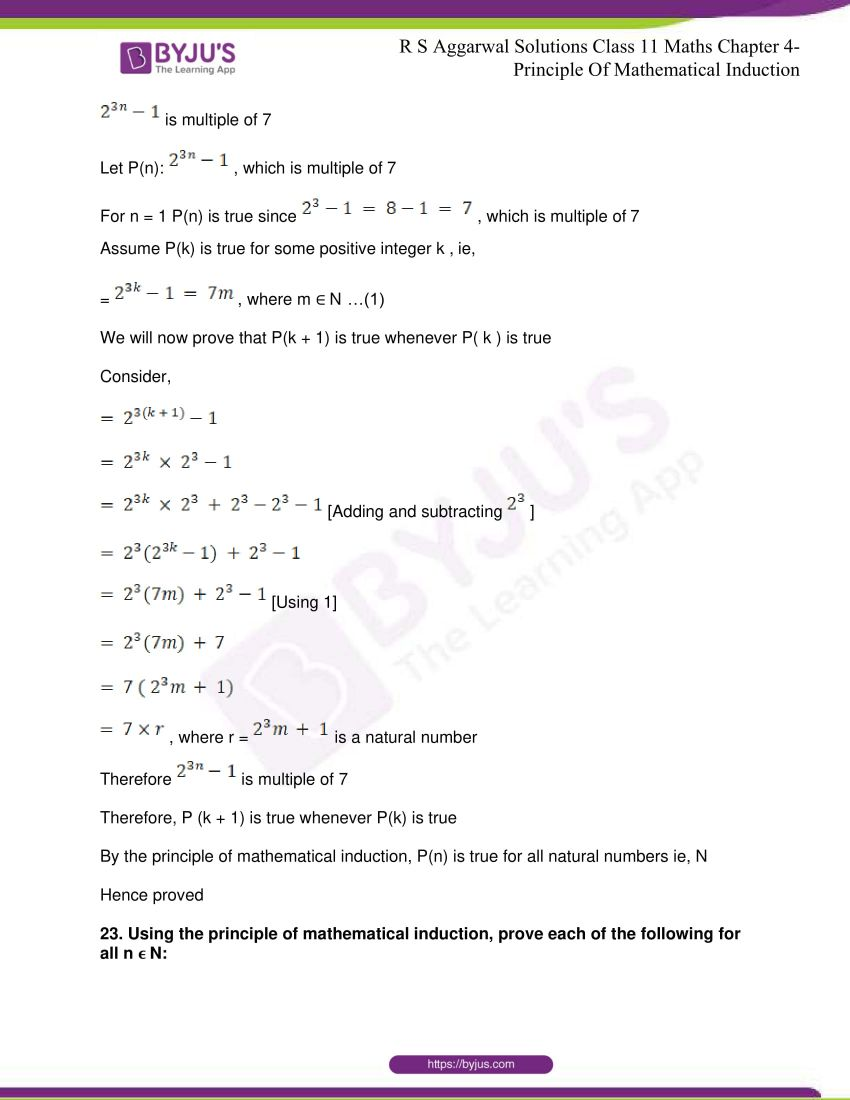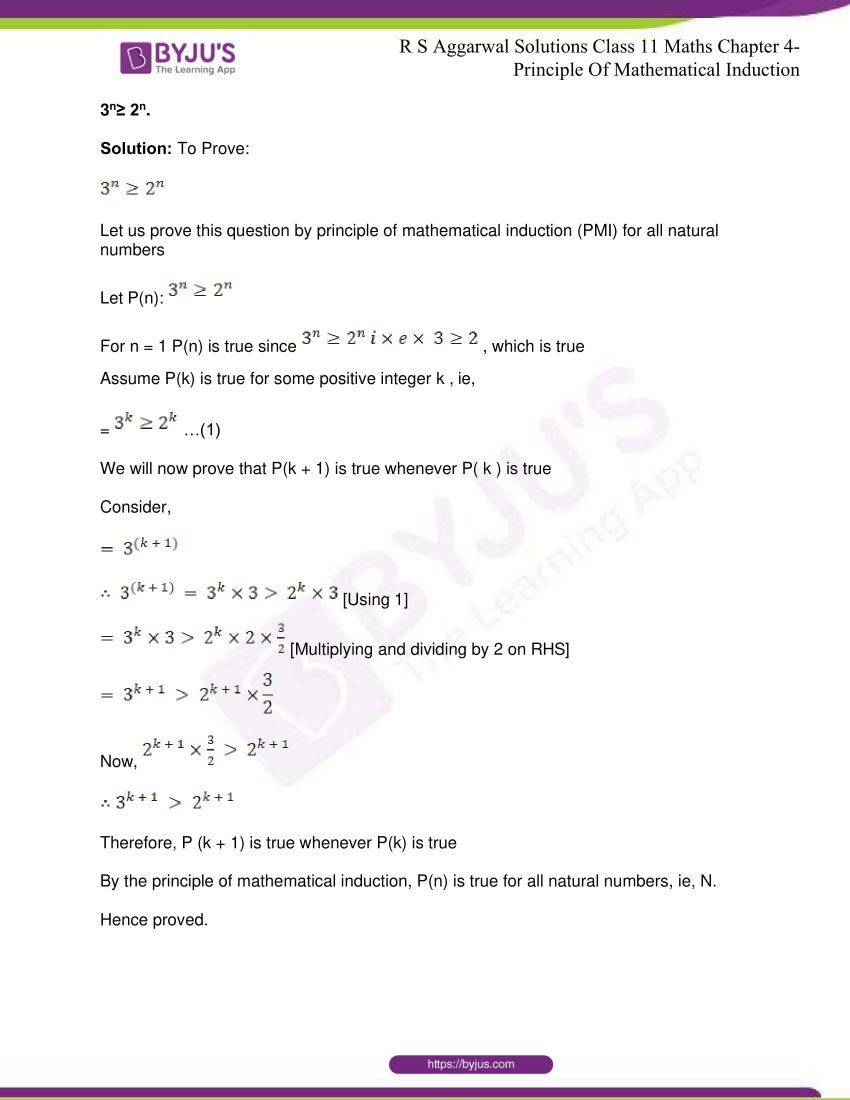## R S Aggarwal Solutions for Class 11 Maths Chapter 4- Principle of Mathematical Induction

Chapter 4, Principle of Mathematical Induction is composed of one exercise and the solutions given here contain answers to all the questions present in this exercise. Different problems related to the Principle of Mathematical Induction are being discussed in this chapter.

## R S Aggarwal Solutions for Class 11 Maths Chapter 4- Principle of Mathematical Induction

In Chapter 4 of Class 11, the students are taught about the Principle of Mathematical Induction. The chapter revolves around the definition: Let P(n) be a statement involving the natural number n such that (i) P(1) is true and P(k+1) is true, whenever P(k) is true then P(n) is true for all n belonging to N. Study Chapter 4 of R S Aggarwal textbook to understand more about Principle of Mathematical Induction. Learn the R S Aggarwal Solutions for Class 11 effectively to come out with flying colours in the examinations.